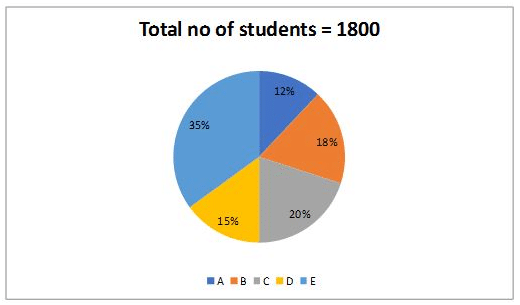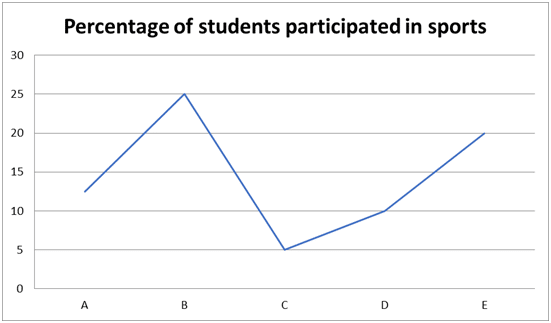# SBI Clerk Mains Quantitative Aptitude (Day-48)

Dear Aspirants, Our IBPS Guide team is providing new series of Quantitative Aptitude Questions for SBI Clerk Mains 2020 so the aspirants can practice it on a daily basis. These questions are framed by our skilled experts after understanding your needs thoroughly. Aspirants can practice these new series questions daily to familiarize with the exact exam pattern and make your preparation effective.

Start Quiz

Missing Number series

Directions (01 – 05): The questions below are based on the given series I. The series I satisfies a certain pattern. Follow the same pattern in series II and answer the questions.

1)

I). 72, 182, 272, 344, 400, 442

II). 175……545

If 545 is the nth term, then find the value of n?

A) 8

B) 4

C) 7

D) 5

E) 6

2)

I). 201, 180, 161, 145, 134, 130

II). 516, 500……469

If 469 is the nth term, then what value should come in (n – 2)th term?

A) 474

B) 486

C) 475

D) 476

E) 485

3)

I). 165, 165, 660, 10560, 675840

II). 210…….840

If 840 is the 3rd term, then what value should come in 5th term?

A) 860360

B) 135400

C) 860260

D) 134440

E) 860160

4)

I). 10, 15, 40, 135, 560, 2825

II). 25, _, _, _, _, ?

What value should come at the place of question mark?

A) 4225

B) 4705

C) 4725

D) 4605

E) 4625

5)

I). 700, 635, 583, 544, 518, 505

II). 1196, _, _, _,? ,_.

What value should come at the place of question mark?

A) 1024

B) 1014

C) 1040

D) 1034

E) 1114

Data Interpretation

Directions (06 – 10): Study the following information carefully and answer the questions given below.

The following Pie chart shows the percentage of students in each class and line graph shows the percentage of students participated in sports.6) What is the ratio of the number of students from E to the students participated in sports from C & D?

A) 14: 1

B) 1: 14

C) 13: 21

D) 21: 13

E) None of these

7) What % is the total number of students participated in sports from all the classes together?

A) 15.5%

B) 17.5%

C) 20.5%

D) 13.5%

E) None of these

8) Students participating in sports from C & D together is how much % less than the no. of students in A?

A) 71.72%

B) 73.15%

C) 79.16%

D) 76.18%

E) None of these

9) What is the difference between the no. of students participating sports in A & B together and the total no. of students in D & E together?

A) 792

B) 996

C) 874

D) 926

E) None of these

10) In which of the following school, the no. of students participating in sports is higher?

A) A

B) E

C) B

D) C

E) None of these

Directions (1-5) :

Series I:

72 + (10+ 10) = 182

182 + (9+ 9) = 272

272 + (8+ 8) = 344

344 + (7+ 7) = 400

400 + (6+ 6) = 442

(Or)

The difference of difference is, 20, 18, 16, 14,….

Series II:

175 + (10+ 10) = 285

285 + (9+ 9) = 375

375 + (8+ 8) = 447

447 + (7+ 7) = 503

503 + (6+ 6) = 545

175 is the 1st term, so 545 is the 6th term.

Series I:

Difference of difference is a prime number

201                  180                  161                  145                  134            130

21                    19                    16                    11                    4

2                     3                      5                      7

Series II:

516                  500                  486                  475                  469

16                      14               11                     6

2                      3                     5

516 is the 1st term and 469 is the 5th term

(n – 2) = (5 – 2) = 3rd term = 486

Series I:

165 * 4=165

165 * 4=660

660 * 4=10560

10560 * 4=675840

Series II:

210 * 4= 210

210 * 4= 840

840 * 4= 13440

13440 * 4= 860160

Series I:

(10 + 5) * 1 = 15

(15 + 5) * 2 = 40

(40 + 5) * 3 = 135

(135 + 5) * 4 = 560

(560 + 5) * 5 = 2825

Series II:

(25 + 5) * 1 = 30

(30 + 5) * 2 = 70

(70 + 5) * 3 = 225

(225 + 5) * 4 = 920

(920 + 5) * 5 = 4625

Series I:

700 – 65 = 635

635 – 52 = 583

583 – 39 = 544

544 – 26 = 518

518 – 13 = 505

Series II:

1196 – 65 = 1131

1131 – 52 = 1079

1079 – 39 = 1040

1040 – 26 = 1014

Directions (06 – 10):Required ratio = 630: 45 => 126: 9 => 14: 1

Required percentage = 279/1800 * 100 = 15.5%

Required percentage = [(18 + 27) – 216]/216 * 100

= 171/216 * 100

= 79.16%

Required difference = 900 – 108 = 792

A – 27

B – 81

C – 18

D – 27

E – 126

Class E has maximum number of students participating in sports.

 Check Here to View SBI Clerk Mains 2020 Quantitative Aptitude Questions Day 47 Day 46 Day 45 Click Here for SBI Clerk 2020 – Detailed Exam Notification